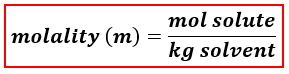# Problem: The density of a 20.3 M CH 3OH (methanol) solution is 0.858 g/mL. What is the molality of this solution? H2O is the solvent. a) 20.8 m b) 97.8 m c) 17.4 m d) 23.7 m e) 70.0 m

###### FREE Expert Solution
88% (275 ratings)
###### FREE Expert Solution

We’re being asked to calculate for the molality of a 20.3 M methanol (CH3OH) solution.

When calculating for molality, we use the following equation:We will calculate the molality of the solution using the following steps:

Step 1: Calculate the mass of the solute.

Step 2: Calculate the mass of the solution.

Step 3: Calculate the mass of the solvent (in kg).

Step 4: Calculate the molality of the solution.

Solute → CH3OH

Solvent → water (H2O)

88% (275 ratings)###### Problem Details

The density of a 20.3 M CH 3OH (methanol) solution is 0.858 g/mL. What is the molality of this solution? H2O is the solvent.

a) 20.8 m

b) 97.8 m

c) 17.4 m

d) 23.7 m

e) 70.0 m

What scientific concept do you need to know in order to solve this problem?

Our tutors have indicated that to solve this problem you will need to apply the Molality concept. You can view video lessons to learn Molality. Or if you need more Molality practice, you can also practice Molality practice problems.

What is the difficulty of this problem?

Our tutors rated the difficulty ofThe density of a 20.3 M CH 3OH (methanol) solution is 0.858 ...as medium difficulty.

How long does this problem take to solve?

Our expert Chemistry tutor, Sabrina took 7 minutes and 9 seconds to solve this problem. You can follow their steps in the video explanation above.

What professor is this problem relevant for?

Based on our data, we think this problem is relevant for Professor Bryant's class at USF.